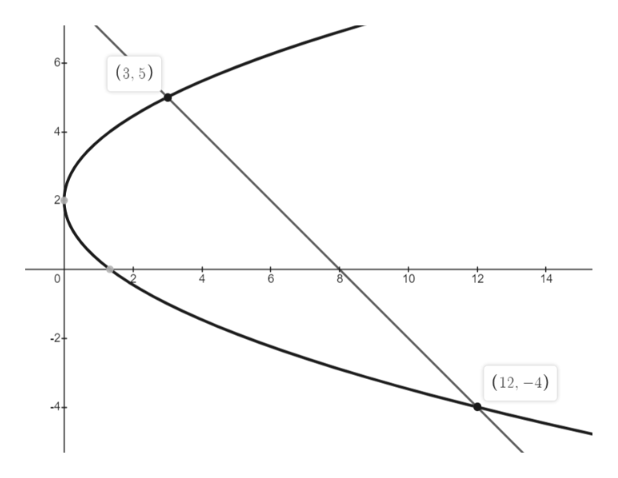After working an integration problem for the area formed by two intersecting line functions (8-y) - (y-2)2/3 dy  between -4 and 5 in which I let u subsitition equal the (y-2)2/3 as follows: A = intergration from -4 to 5 (correctly checked) of (8-y) - ( u ) dy  gave an answer that was positive but did not match the book answer of 81/2. My calculation for (dy ) was as follows:  du = (y2 - 4y + 4)/3  dy. Since I am using this problem as a model to my understanding of Briggs Calculus chapter 6.2, 3rd ed., would you give me a step by step solution to this problem to help me understand the nature of my error(s)?

Question

After working an integration problem for the area formed by two intersecting line functions (8-y) - (y-2)2/3 dy  between -4 and 5 in which I let u subsitition equal the (y-2)2/3 as follows: A = intergration from -4 to 5 (correctly checked) of (8-y) - ( u ) dy  gave an answer that was positive but did not match the book answer of 81/2.

My calculation for (dy ) was as follows:  du = (y2 - 4y + 4)/3  dy.

Since I am using this problem as a model to my understanding of Briggs Calculus chapter 6.2, 3rd ed., would you give me a step by step solution to this problem to help me understand the nature of my error(s)?

Step 1

The given two intersecting line function is ((8–y)–(y–2)2/3) dy.

Plot the graph of two lines a...help_outlineImage Transcriptionclose6- (3,5) 10 12 14 (12, -4) 2 fullscreen

Want to see the full answer?

See Solution

Want to see this answer and more?

Our solutions are written by experts, many with advanced degrees, and available 24/7

See Solution
Tagged in

Integration